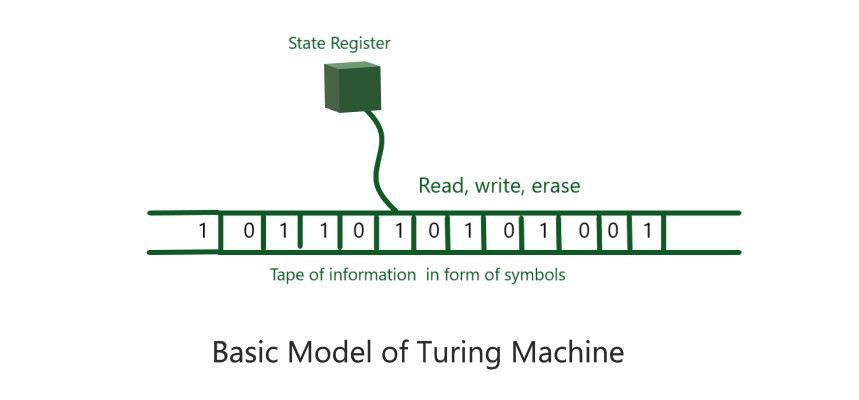# Origin of Computer Science

• Difficulty Level : Easy
• Last Updated : 30 Sep, 2021

Overview :
Computer Science is the science of computers how computers work and do computations. Laptops, desktops, quantum computers, microprocessors, etc. are all the physical aspects of a computer, but there are also theoretical aspects like algorithms, software, computability, complexity theory etc. But have you ever wondered what led to the discovery of computer science?

Formal System :
Computer Science is a result of applied mathematics as it was created from trying to answer very abstract questions about the foundation of Mathematics. Back in the 1900s, mathematics did not have certain fixed rules. The philosophers proposed that mathematical objects, shapes, numbers and the relationships between them belong to their ideal world and referred to it as the ‘world of forms’  and referred to mathematics as a mental construct and all gave different intuitions about Mathematics. But the German mathematician David Hilbert proposed to axiomatize all of mathematics. He proposed that Mathematics should be treated as a Formal System. A Formal system is an alphabet or set of symbols which can be manipulated with a set of rules for ex-chess.

Concepts :
The 3 main concepts that Hilbert put up in the Foundational System of Mathematics were as follows.

1. Consistency –
No contradictions can be proven within the system.

2. Completeness-
A proof was required that all true mathematical statements which existed within the system could be proven within the system.

3. Decidability-
An effective procedure for deciding the truth or falsity of any mathematical statement. Decidability is one of the fascinating concept which laid the foundation of computation and computer science.

Turing Test :
The famous Mathematician Alan Turing (also known as the father of Computer science) tried to understand the question of decidability deeper and thought that “Did there exist an effective procedure for deciding the truth or falsity of any mathematical statement.” Throughout his life, Turing was fascinated with the relationship between humans and machines. He came up with the most revolutionizing idea which he named the Turing Test- a test for determining whether a machine is capable of thinking like a human and defined the term effective procedures. He thought about the basic components of what humans do while computing as follows.